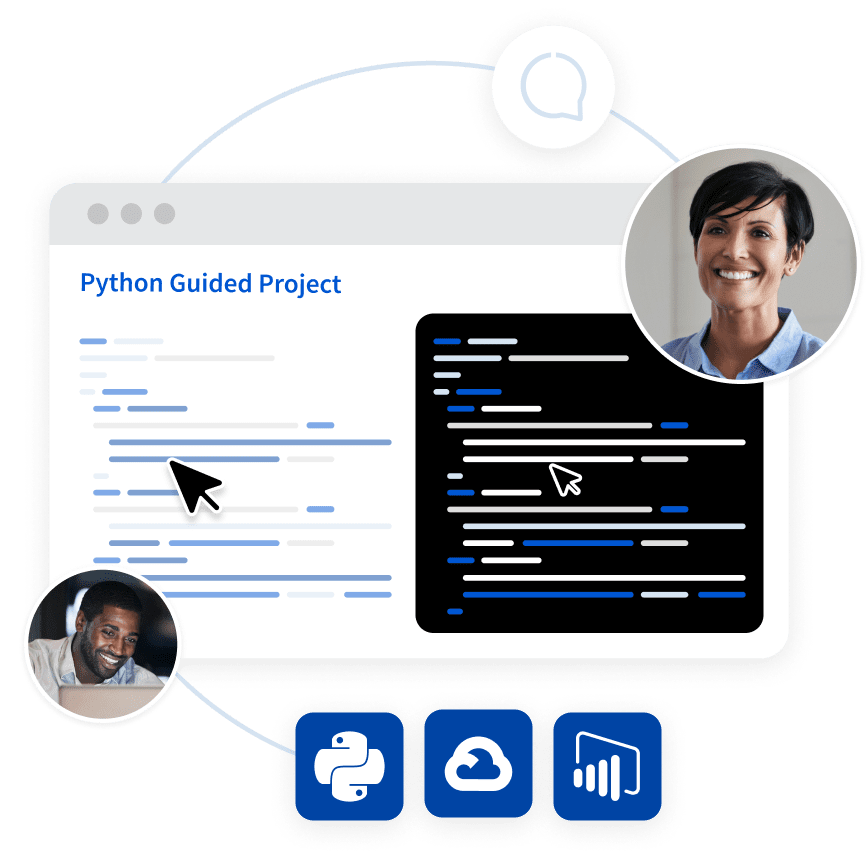## Python Tutorial

Python HOME Python Intro Python Get Started Python Syntax Python Comments Python Variables Python Data Types Python Numbers Python Casting Python Strings Python Booleans Python Operators Python Lists Python Tuples Python Sets Python Dictionaries Python If...Else Python While Loops Python For Loops Python Functions Python Lambda Python Arrays Python Classes/Objects Python Inheritance Python Iterators Python Scope Python Modules Python Dates Python Math Python JSON Python RegEx Python PIP Python Try...Except Python User Input Python String Formatting

## File Handling

Python File Handling Python Read Files Python Write/Create Files Python Delete Files

## Python Modules

NumPy Tutorial Pandas Tutorial SciPy Tutorial Django Tutorial

## Python Matplotlib

Matplotlib Intro Matplotlib Get Started Matplotlib Pyplot Matplotlib Plotting Matplotlib Markers Matplotlib Line Matplotlib Labels Matplotlib Grid Matplotlib Subplot Matplotlib Scatter Matplotlib Bars Matplotlib Histograms Matplotlib Pie Charts

## Machine Learning

Getting Started Mean Median Mode Standard Deviation Percentile Data Distribution Normal Data Distribution Scatter Plot Linear Regression Polynomial Regression Multiple Regression Scale Train/Test Decision Tree Confusion Matrix Hierarchical Clustering Logistic Regression Grid Search Categorical Data K-means Bootstrap Aggregation Cross Validation AUC - ROC Curve K-nearest neighbors

## Python MySQL

MySQL Get Started MySQL Create Database MySQL Create Table MySQL Insert MySQL Select MySQL Where MySQL Order By MySQL Delete MySQL Drop Table MySQL Update MySQL Limit MySQL Join

## Python MongoDB

MongoDB Get Started MongoDB Create Database MongoDB Create Collection MongoDB Insert MongoDB Find MongoDB Query MongoDB Sort MongoDB Delete MongoDB Drop Collection MongoDB Update MongoDB Limit

## Python Reference

Python Overview Python Built-in Functions Python String Methods Python List Methods Python Dictionary Methods Python Tuple Methods Python Set Methods Python File Methods Python Keywords Python Exceptions Python Glossary

## Module Reference

Random Module Requests Module Statistics Module Math Module cMath Module

## Python How To

Remove List Duplicates Reverse a String Add Two Numbers

## Python Examples

Python Examples Python Compiler Python Exercises Python Quiz Python Certificate

# Machine Learning

Machine Learning is making the computer learn from studying data and statistics.

Machine Learning is a step into the direction of artificial intelligence (AI).

Machine Learning is a program that analyses data and learns to predict the outcome.

## Where To Start?

In this tutorial we will go back to mathematics and study statistics, and how to calculate important numbers based on data sets.

We will also learn how to use various Python modules to get the answers we need.

And we will learn how to make functions that are able to predict the outcome based on what we have learned.

## Data Set

In the mind of a computer, a data set is any collection of data. It can be anything from an array to a complete database.

Example of an array:

`[99,86,87,88,111,86,103,87,94,78,77,85,86]`

Example of a database:

 Carname Color Age Speed AutoPass BMW red 5 99 Y Volvo black 7 86 Y VW gray 8 87 N VW white 7 88 Y Ford white 2 111 Y VW white 17 86 Y Tesla red 2 103 Y BMW black 9 87 Y Volvo gray 4 94 N Ford white 11 78 N Toyota gray 12 77 N VW white 9 85 N Toyota blue 6 86 Y

By looking at the array, we can guess that the average value is probably around 80 or 90, and we are also able to determine the highest value and the lowest value, but what else can we do?

And by looking at the database we can see that the most popular color is white, and the oldest car is 17 years, but what if we could predict if a car had an AutoPass, just by looking at the other values?

That is what Machine Learning is for! Analyzing data and predicting the outcome!

In Machine Learning it is common to work with very large data sets. In this tutorial we will try to make it as easy as possible to understand the different concepts of machine learning, and we will work with small easy-to-understand data sets.

## Learn to Filter Data in Python Like a Data AnalystTry a hands-on training sessions with step-by-step guidance from an expert. Try the guided project made in collaboration with Coursera now!

Get started

## Data Types

To analyze data, it is important to know what type of data we are dealing with.

We can split the data types into three main categories:

• Numerical
• Categorical
• Ordinal

Numerical data are numbers, and can be split into two numerical categories:

• Discrete Data
- numbers that are limited to integers. Example: The number of cars passing by.
• Continuous Data
- numbers that are of infinite value. Example: The price of an item, or the size of an item

Categorical data are values that cannot be measured up against each other. Example: a color value, or any yes/no values.

Ordinal data are like categorical data, but can be measured up against each other. Example: school grades where A is better than B and so on.

By knowing the data type of your data source, you will be able to know what technique to use when analyzing them.